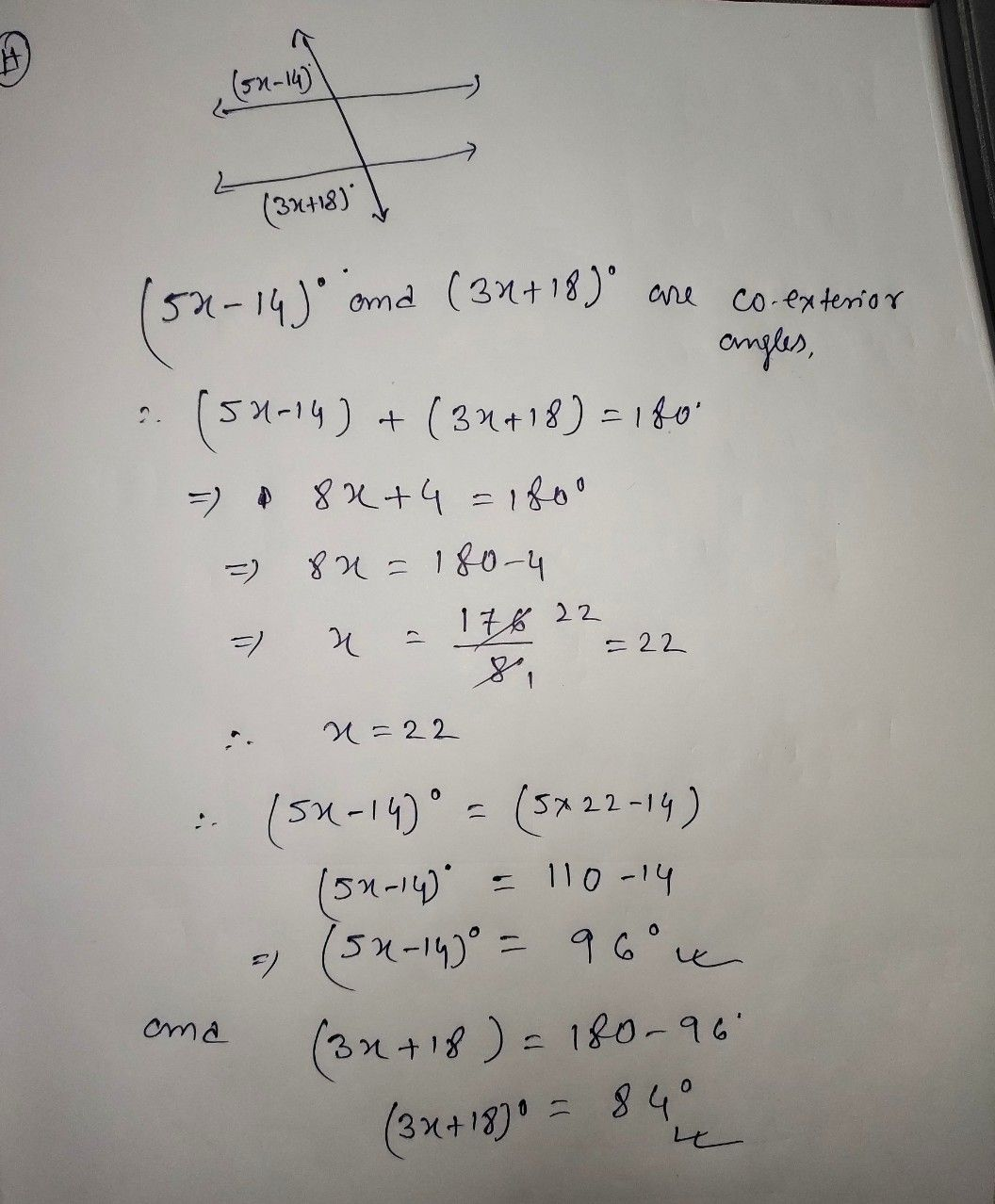Symbol
Problem$4$ $\left(5x→14\right)^{0}1$ $1$ $4$ $\left(3x+18\right)^{°}$ $1$
10th-13th grade
Geometry
SolutionQanda teacher - DEBOif you have any question about my answer then please ask me